# Visualizations and Animations for Agent Based Models

This page describes functions that can be used in conjunction with Agents.jl to animate and interact with agent based models.

The animation at the start of the page is created using the code of this page, see below.

The docs are built using versions:

using Pkg
Pkg.status(["Agents", "InteractiveDynamics", "CairoMakie"];
mode = PKGMODE_MANIFEST, io=stdout
)
      Status ~/work/Agents.jl/Agents.jl/docs/Manifest.toml
[46ada45e] Agents v5.4.3 ~/work/Agents.jl/Agents.jl
[13f3f980] CairoMakie v0.8.12
[ec714cd0] InteractiveDynamics v0.21.10

## Static plotting of ABMs

Static plotting, which is also the basis for creating custom plots that include an abm plot, is done using the abmplot function. Its usage is exceptionally straight-forward, and in principle one simply defines functions for how the agents should be plotted. Here we will use a pre-defined model, the Daisyworld as an example throughout this docpage. To learn about this model you can visit the full example,

using InteractiveDynamics, Agents
using CairoMakie
daisypath = joinpath(dirname(pathof(InteractiveDynamics)), "agents", "daisyworld_def.jl")
include(daisypath)
model, daisy_step!, daisyworld_step! = daisyworld(;
solar_luminosity = 1.0, solar_change = 0.0, scenario = :change
)
model
AgentBasedModel with 360 agents of type Daisy
space: GridSpaceSingle with size (30, 30), metric=chebyshev, periodic=true
scheduler: fastest
properties: temperature, solar_luminosity, max_age, surface_albedo, ratio, solar_change, tick, scenario

Now, to plot daisyworld is as simple as

daisycolor(a::Daisy) = a.breed # color of agents
as = 20 # size of agents
am = '✿' # marker of agents
scatterkwargs = (strokewidth = 1.0,) # add stroke around each agent
fig, ax, abmobs = abmplot(model; ac = daisycolor, as, am, scatterkwargs)
fig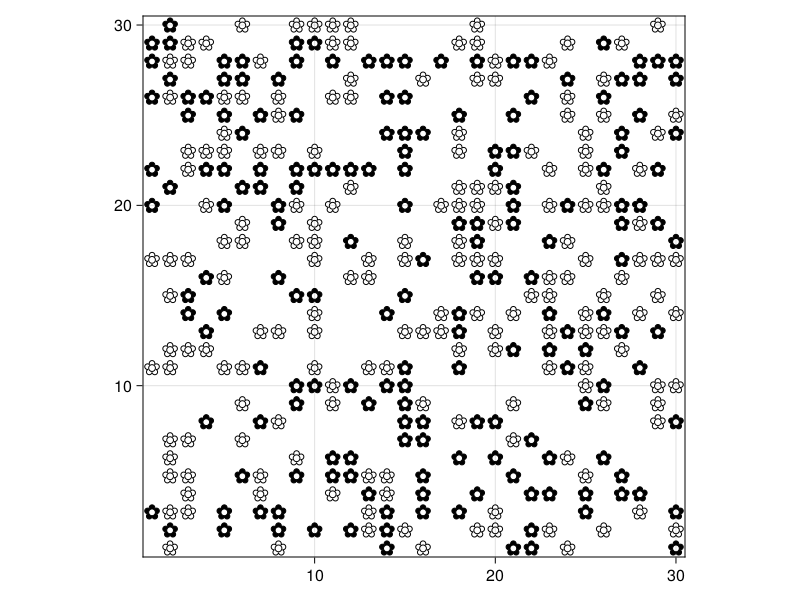To this, we can also plot the temperature of the planet by providing the access field as a heat array:

heatarray = :temperature
heatkwargs = (colorrange = (-20, 60), colormap = :thermal)
plotkwargs = (;
ac = daisycolor, as, am,
scatterkwargs = (strokewidth = 1.0,),
heatarray, heatkwargs
)

fig, ax, abmobs = abmplot(model; plotkwargs...)
fig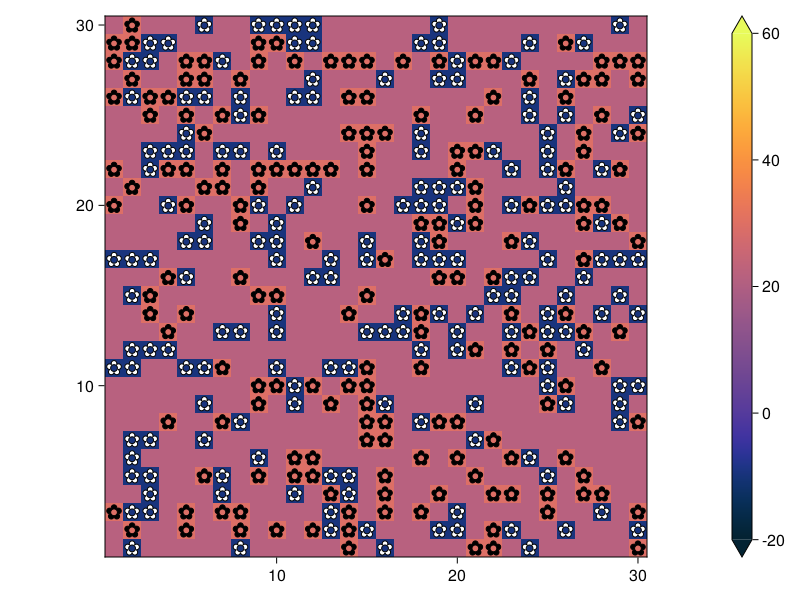InteractiveDynamics.abmplotFunction
abmplot(model::ABM; kwargs...) → fig, ax, abmobs
abmplot!(ax::Axis/Axis3, model::ABM; kwargs...) → abmobs

Plot an agent based model by plotting each individual agent as a marker and using the agent's position field as its location on the plot. The same function is used to make custom composite plots and interactive applications for the model evolution using the returned abmobs. abmplot is also used to launch interactive GUIs for evolving agent based models, see "Interactivity" below.

Requires Agents. See also abmvideo and abmexploration.

Keyword arguments

Agent related

• ac, as, am : These three keywords decide the color, size, and marker, that each agent will be plotted as. They can each be either a constant or a function, which takes as an input a single agent and outputs the corresponding value.

Using constants: ac = "#338c54", as = 15, am = :diamond

Using functions:

ac(a) = a.status == :S ? "#2b2b33" : a.status == :I ? "#bf2642" : "#338c54"
as(a) = 10rand()
am(a) = a.status == :S ? :circle : a.status == :I ? :diamond : :rect

Notice that for 2D models, am can be/return a Polygon instance, which plots each agent as an arbitrary polygon. It is assumed that the origin (0, 0) is the agent's position when creating the polygon. In this case, the keyword as is meaningless, as each polygon has its own size. Use the functions scale, rotate2D to transform this polygon.

3D models currently do not support having different markers. As a result, am cannot be a function. It should be a Mesh or 3D primitive (such as Sphere or Rect3D).

• offset = nothing : If not nothing, it must be a function taking as an input an agent and outputting an offset position tuple to be added to the agent's position (which matters only if there is overlap).

• scatterkwargs = () : Additional keyword arguments propagated to the scatter! call.

Preplot related

• heatarray = nothing : A keyword that plots a heatmap over the space. Its values can be standard data accessors given to functions like run!, i.e. either a symbol (directly obtain model property) or a function of the model. The returned data must be a matrix of the same size as the underlying space. For example heatarray = :temperature is used in the Daisyworld example. But you could also define f(model) = create_matrix_from_model... and set heatarray = f. The heatmap will be updated automatically during model evolution in videos and interactive applications.

It is strongly recommended to use abmplot instead of the abmplot! method if you use heatarray, so that a colorbar can be placed naturally.

• heatkwargs = NamedTuple() : Keywords given to Makie.heatmap function if heatarray is not nothing.

• add_colorbar = true : Whether or not a Colorbar should be added to the right side of the heatmap if heatarray is not nothing.

• static_preplot! : A function f(ax, model) that plots something after the heatmap but before the agents.

• osmkwargs = NamedTuple() : keywords directly passed to osmplot! from OSMMakie.jl if model space is OpenStreetMapSpace.

The stand-alone function abmplot also takes two optional NamedTuples named figure and axis which can be used to change the automatically created Figure and Axis objects.

Interactivity

Evolution related

• agent_step!, model_step! = Agents.dummystep: Stepping functions to pass to ABMObservable which itself passes to Agents.step!.
• add_controls::Bool: If true, abmplot switches to "interactive application" mode. This is by default true if either agent_step! or model_step! keywords are provided. These stepping functions are used to evolve the model interactively using Agents.step!. The application has the following interactive elements:
1. "step": advances the simulation once for spu steps.
2. "run": starts/stops the continuous evolution of the model.
3. "reset model": resets the model to its initial state from right after starting the interactive application.
4. Two sliders control the animation speed: "spu" decides how many model steps should be done before the plot is updated, and "sleep" the sleep() time between updates.
• enable_inspection = add_controls: If true, enables agent inspection on mouse hover.
• spu = 1:50: The values of the "spu" slider.
• params = Dict() : This is a dictionary which decides which parameters of the model will be configurable from the interactive application. Each entry of params is a pair of Symbol to an AbstractVector, and provides a range of possible values for the parameter named after the given symbol (see example online). Changing a value in the parameter slides is only propagated to the actual model after a press of the "update" button.

Data collection related

• adata, mdata, when: Same as the keyword arguments of Agents.run!. If either or both adata, mdata are given, data are collected and stored in the abmobs, see ABMObservable. The same keywords provide the data plots of abmexploration. This also adds the button "clear data" which deletes previously collected agent and model data by emptying the underlying DataFrames adf/mdf. Reset model and clear data are independent processes.

See the documentation string of ABMObservable for custom interactive plots.

## Interactive ABM Applications

Continuing from the Daisyworld plots above, we can turn them into interactive applications straightforwardly, simply by providing the stepping functions as illustrated in the documentation of abmplot. Note that GLMakie should be used instead of CairoMakie when wanting to use the interactive aspects of the plots.

fig, ax, abmobs = abmplot(model;
agent_step! = daisy_step!, model_step! = daisyworld_step!,
plotkwargs...)
fig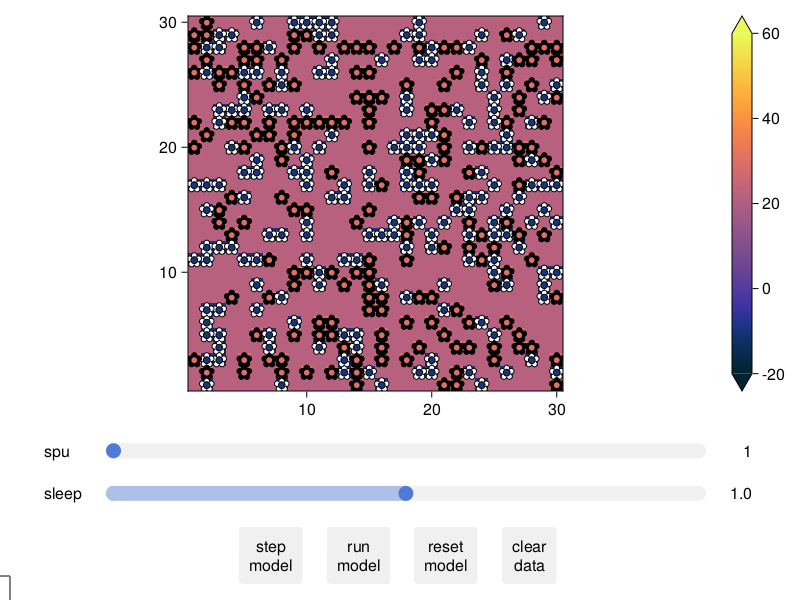One could click the run button and see the model evolve. Furthermore, one can add more sliders that allow changing the model parameters.

params = Dict(
:surface_albedo => 0:0.01:1,
:solar_change => -0.1:0.01:0.1,
)
fig, ax, abmobs = abmplot(model;
agent_step! = daisy_step!, model_step! = daisyworld_step!,
params, plotkwargs...)
fig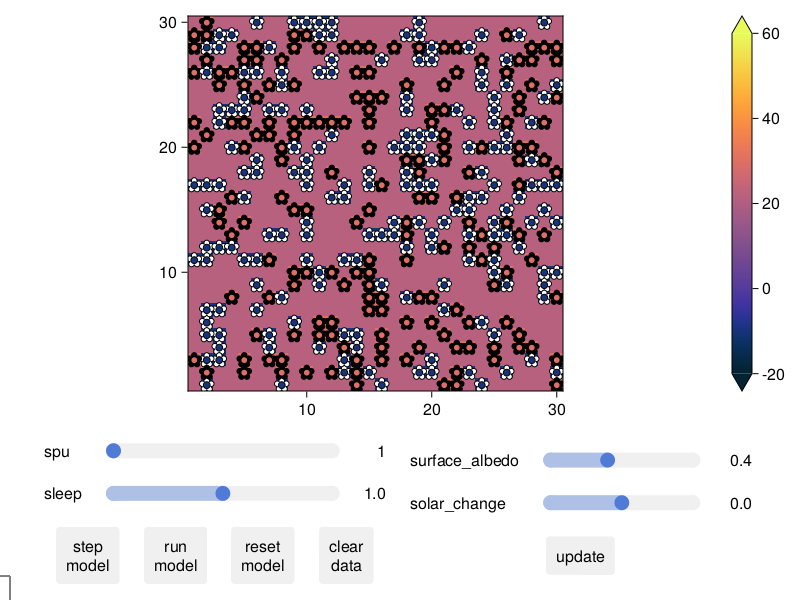One can furthermore collect data while the model evolves and visualize them using the convenience function abmexploration

using Statistics: mean
black(a) = a.breed == :black
white(a) = a.breed == :white
adata = [(black, count), (white, count)]
temperature(model) = mean(model.temperature)
mdata = [temperature, :solar_luminosity]
fig, abmobs = abmexploration(model;
agent_step! = daisy_step!, model_step! = daisyworld_step!, params, plotkwargs...,
adata, alabels = ["Black daisys", "White daisys"], mdata, mlabels = ["T", "L"]
)
InteractiveDynamics.abmexplorationFunction
abmexploration(model::ABM; alabels, mlabels, kwargs...)

Open an interactive application for exploring an agent based model and the impact of changing parameters on the time evolution. Requires Agents.

The application evolves an ABM interactively and plots its evolution, while allowing changing any of the model parameters interactively and also showing the evolution of collected data over time (if any are asked for, see below). The agent based model is plotted and animated exactly as in abmplot, and the model argument as well as splatted kwargs are propagated there as-is. This convencience function only works for aggregated agent data.

Calling abmexploration returns: fig::Figure, p::_ABMPlot. So you can save and/or further modify the figure. But it is also possible to access the collected data (if any) via the plot object, just like in the case of using abmplot directly.

Clicking the "reset" button will add a red vertical line to the data plots for visual guidance.

Keywords arguments (in addition to those in abmplot)

• alabels, mlabels: If data are collected from agents or the model with adata, mdata, the corresponding plots' y-labels are automatically named after the collected data. It is also possible to provide alabels, mlabels (vectors of strings with exactly same length as adata, mdata), and these labels will be used instead.
• figure = NamedTuple(): Keywords to customize the created Figure.
• axis = NamedTuple(): Keywords to customize the created Axis.
• plotkwargs = NamedTuple(): Keywords to customize the styling of the resulting scatterlines plots.

## ABM Videos

InteractiveDynamics.abmvideoFunction
abmvideo(file, model, agent_step! [, model_step!]; kwargs...)

This function exports the animated time evolution of an agent based model into a video saved at given path file, by recording the behavior of the interactive version of abmplot (without sliders). The plotting is identical as in abmplot and applicable keywords are propagated.

Keywords

• spf = 1: Steps-per-frame, i.e. how many times to step the model before recording a new frame.
• framerate = 30: The frame rate of the exported video.
• frames = 300: How many frames to record in total, including the starting frame.
• title = "": The title of the figure.
• showstep = true: If current step should be shown in title.
• figure = NamedTuple(): Figure related keywords (e.g. resolution, backgroundcolor).
• axis = NamedTuple(): Axis related keywords (e.g. aspect).
• recordkwargs = NamedTuple(): Keyword arguments given to Makie.record. You can use (compression = 1, profile = "high") for a higher quality output, and prefer the CairoMakie backend. (compression 0 results in videos that are not playable by some software)
• kwargs...: All other keywords are propagated to abmplot.

E.g., continuing from above,

model, daisy_step!, daisyworld_step! = daisyworld()
abmvideo(
"daisyworld.mp4",
model,  daisy_step!, daisyworld_step!;
title = "Daisy World", frames = 150,
plotkwargs...
)

## Agent inspection

It is possible to inspect agents at a given position by hovering the mouse cursor over the scatter points in the agent plot. Inspection is automatically enabled for interactive applications (i.e. when either agent or model stepping functions are provided). To manually enable this functionality, simply add enable_inspection = true as an additional keyword argument to the abmplot/abmplot! call. A tooltip will appear which by default provides the name of the agent type, its id, pos, and all other fieldnames together with their current values. This is especially useful for interactive exploration of micro data on the agent level.The tooltip can be customized by extending InteractiveDynamics.agent2string.

InteractiveDynamics.agent2stringFunction
agent2string(agent::A)

Convert agent data into a string which is used to display all agent variables and their values in the tooltip on mouse hover. Concatenates strings if there are multiple agents at one position. Custom tooltips for agents can be implemented by adding a specialised method for agent2string. Example:

function InteractiveDynamics.agent2string(agent::SpecialAgent)
"""
✨ SpecialAgent ✨
ID = $(agent.id) Main weapon =$(agent.charisma)
Side weapon = \$(agent.pistol)
"""
end

## Creating custom ABM plots

The existing convenience function abmexploration will always display aggregated collected data as scatterpoints connected with lines. In cases where more granular control over the displayed plots is needed, we need to take a few extra steps and utilize the ABMObservable returned by abmplot.

InteractiveDynamics.ABMObservableType
ABMObservable(model; agent_step!, model_step!, adata, mdata, when) → abombs

abmobs contains all information necessary to step an agent based model interactively. It is also returned by abmplot.

Calling Agents.step!(abmobs, n) will step the model for n using the provided agent_step!, model_step!, n as in Agents.step!.

The fields abmobs.model, abmobs.adf, abmobs.mdf are observables that contain the AgentBasedModel, and the agent and model dataframes with collected data. Data are collected as described in Agents.run! using the adata, mdata, when keywords. All three observables are updated on stepping (when it makes sense). The field abmobs.s is also an observable containing the current step number.

All plotting and interactivity should be defined by lifting these observables.

To do custom animations you need to have a good idea of how Makie's animation system works. Have a look at this tutorial if you are not familiar yet.

create a basic abmplot with controls and sliders

model, = daisyworld(; solar_luminosity = 1.0, solar_change = 0.0, scenario = :change)
fig, ax, abmobs = abmplot(model;
agent_step! = daisy_step!, model_step! = daisyworld_step!, params, plotkwargs...,
adata, mdata, figure = (; resolution = (1600,800))
)
fig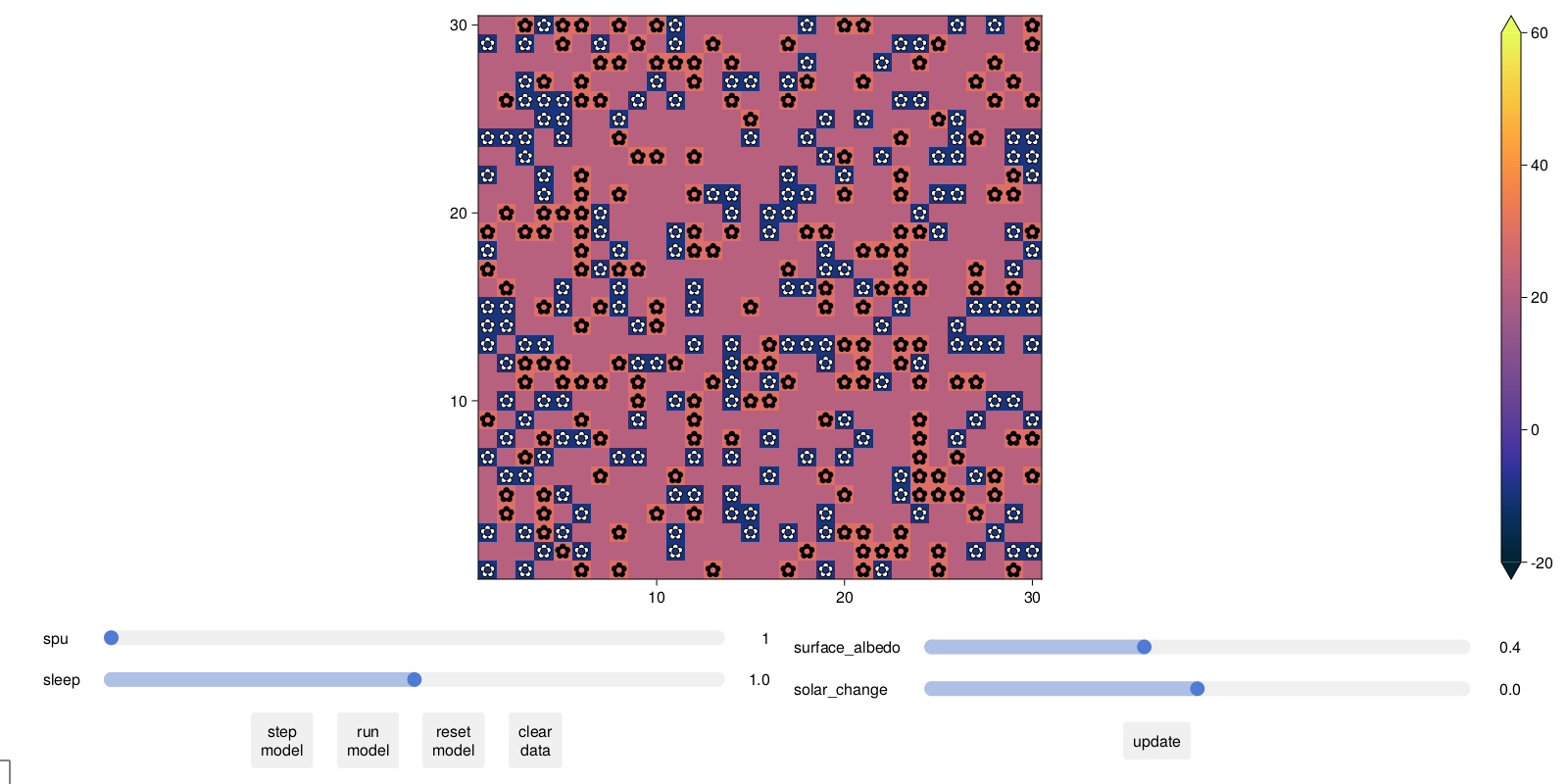abmobs
ABMObservable with model:
AgentBasedModel with 360 agents of type Daisy
space: GridSpaceSingle with size (30, 30), metric=chebyshev, periodic=true
scheduler: fastest
properties: temperature, solar_luminosity, max_age, surface_albedo, ratio, solar_change, tick, scenario
and with data collection:
adata: Tuple{Function, typeof(count)}[(Main.ex-agents_visualizations.black, count), (Main.ex-agents_visualizations.white, count)]
mdata: Any[Main.ex-agents_visualizations.temperature, :solar_luminosity]
# create a new layout